# Variables in C++

## Variables in C++ with Examples:

In this article, I am going to discuss Variables in C++ with Examples. Please read our previous article where we discussed Primitive Data Types in C++ with Examples. At the end of this article, you will learn everything about C++ Variables with Examples.

##### Variables in C++:

Variables are the names given to data. Variables have data types. The Variable is the place where we store the data in our program. If we have data then we can process the data. So, we require variables to store the data so that we can perform operations on that data.

In mathematics, we have also the concept of variables, so from there, we got the idea to store data in variables.

Variables are the name given to the memory location where we store the data. Variable has the same meaning as that of maths. x-5=0 here x is a variable whose value is 5 means x is nothing but 5 or x is equal to 5. Similarly, in the C++ variable is used to store the values it may be an integer value, character value, or anything.

Ax+bx+c=0 in math, here only x is considered as variable, and other is considered as constants similar to qualify a variable is a valid one or not, to declare a variable there is a rule.

Variables should have data types; which means what type of data we are storing in the variable so that we can perform different operations on different data types. Like addition in integer or float means adding two values mathematically i.e. 7 + 8 = 15 but the addition in a string means combining two different words or sentences into one i.e. “Ram” + “Kumar” = “Ram Kumar”. So that’s why we need to store the data accordingly to our condition of operations which we want to perform on the data.

##### How to use variables in C++?

So let us see how to use variables. Suppose we want to store the ID of a student. So, ID is of integer type, so we will take a variable of int type: int ID; This is the method of declaring a variable. ‘int’ is the data type and ‘ID’ is the variable name. We have to declare variables before using them in the program.

Every variable must be declared then it will be used. At runtime, the variable ‘ID’ will consume 2 bytes of memory. We are considering here the int will take 2 bytes or you can take 4 bytes also but here we are taking 2 bytes. We know every byte in the memory has an address.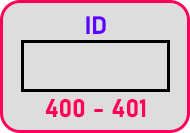These are 2 bytes in which any number we can store in the ‘ID’ variable, and will store in the memory at runtime of the program. Now let us store something in the above-declared variable:

ID = 3422;

Here we have given the value to the ‘ID’ variable. So, 3422 is assigned to the ID variable. Before assigning the value to the variable, there was some garbage value inside the memory of the ID variable. Garbage value means unknown value. Now the number 3422 is stored in the memory.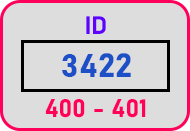Now we can say that the ‘ID’ variable is initialized.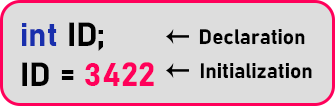First, we have declared a variable, then we have initialized the variable with some value. At runtime, this variable will occupy some space in the memory. Now one more thing is that we can declare and initialize the variable at once like: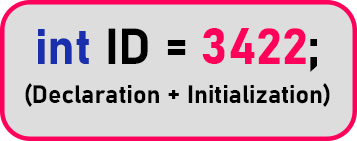This is a declaration as well as initialization. This is how we can declare and initialize variables in C++. Now suppose we want to have a variable to store a section or group, so which type of variable we should take? We will take char data type:We have to remember one thing every time we assign any character to a char type variable, we have to assign it within single quotes as you can see in the above statement. Now if we want to store the price of any item like 4 dollars or 50 cents then we will take float data type to store that type of data.We will define float like this. Here we have to put ‘f’ after the numerical value. We put ‘f’ at the end of the statement because this will indicate that it is a float value. It is not compulsory but it is better to put ‘f’. Because if we don’t write ‘f’ then it will be assumed as the type of double.

So, if you want to write float then write ‘f’ after numerical data or if you want to write double then don’t put the ‘f’. Because float takes 4 bytes and double takes 8 bytes. Double will be initialized as:##### Rules for variable naming in C++:

Now we will discuss the rules for variable naming in C++. We will tell you the valid and invalid names. Let us write that name and we will you why they are valid or invalid.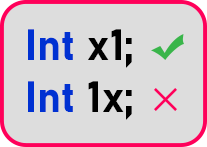x1’ is valid and ‘1x’ is invalid means numerical value, in the beginning, is not allowed.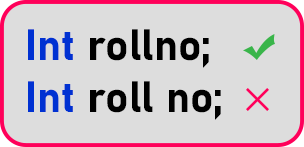Here ‘rollno’ is valid but ‘roll no’ is invalid because space is not allowed in variable naming. If we want to read the word separately then we can add underscore i.e: Int roll_no;

One more way which is more followed in java is int rollNo; This is known as camel notation. Most C++ programmers follow this. The last thing is that when you are giving variable names, don’t give names like x1, x2… Give some meaningful names so that names show what type of value it contains.

###### Valid variables:
1. Variable names should be from 1 to 255 characters in length.
2. A variable name should be beginning with the alphabets or underscore ‘_’.

Example: ash, _ash, a23, A41, A2ds

###### Invalid variables:
1. We cannot use the keyword as a variable name.
2. We cannot use spaces or a special character as a variable name.

Example: int, const, ash wath, ash@sdf! Etc. are examples of invalid variable names.

In the next article, I am going to discuss Arithmetic Operations in C++ with Examples. Here, in this article, I try to explain Variables in C++ with Examples and I hope you enjoy this Variables in C++ with Examples article.